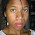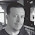## Monday, September 5, 2016

### Retro: A Quick Look at the TI-74 (and HHC 2016)

Retro:  A Quick Look at the TI-74 Basicalc

Back to the 1980s Again

Recently I purchased a Texas Instruments TI-74 Basicalc calculator.  The calculator came in great condition, including manuals and a Mathematics module (and the module).

The TI-74 is a BASIC programming calculator (similar to Hewlett Packard’s HP-71B and HP-75 and Casio’s fx-795P).  The keyboard of the TI-74 is very busy and has a QWERTY keyboard.  The keys are nice but I am not able to type fast on it.  The calculator takes four AAA batteries.  The calculator I got was produced in 1985.  The original cost was around \$130, which was a pretty good price for a basic portable BASIC computer/calculator at the time.HP 71B (top) and TI-74 (bottom).  The 74 is a giant!

The TI-74 is a BASIC programming calculator (similar to Hewlett Packard’s HP-71B and HP-75 and Casio’s fx-795P).  The keyboard of the TI-74 is very busy and has a QWERTY keyboard.  The keys are nice but I am not able to type fast on it.  The calculator takes four AAA batteries.  The calculator I got was produced in 1985.  The original cost was around \$110-\$130, which was a pretty good price for a basic portable BASIC computer/calculator at the time.  The one I got on Amazon, originally sold at Brendle's (a defunct chain store which was based in Elkin, North Carolina)

Calculator Mode vs. BASIC Mode

Modes are switched back and forth with the [ MODE ] toggle key.

Calculator Mode

Like the HP-71B, the TI-74 has two modes: Calculator and BASIC mode.  In Calculator mode, the QWERTY keyboard becomes a scientific calculator, with all the functions stated in blue.  For example, the [ S ] key is the angle change key [ DRG ], while the [ U ] key becomes the square key [ x2 ].  In Calculator mode, the TI-74 operates as an AOS (Algebraic Operating System) calculator.  If have worked with a TI-30Xa or a classic TI-36X Solar, you should be familiar with it.

The Display

The numbers are aligned to the right.  What is neat about the display is that the last function used will also be displayed.  For example:  cos(√19°):

 Keys Screen [DRG] (S) to degrees mode DEG is highlighted 19 19  | [ √x ] (I) 4.358898944 | √x [ cos ] (W) .99710753 | cos

Two Argument Functions

One of the quirks about the Calculator mode is how two argument functions are operated.  For input, you will need to press [ (x,y) ] (the L key).

Combinations and Permutations:  Enter n, press [ (x,y) ] (L), r and press either [ nCr ] (J) for combinations or [ nPr ] (H) for permutations.

Polar to Rectangular:  Enter r, press [ (x,y) ] (L), then θ, [ P>R ] (F).  x is displayed first, press [x<>y] (RUN) to show y.

Rectangular to Polar:  Enter x, press [ (x,y) ] (L), then y, press [ INV ] (SHIFT), [ P>R ] (F).  r is displayed first, press [x<>y] (RUN) to show θ.

Strangely enough, this isn’t the percent change function works.  Enter the new amount, press [Δ%] (SPACE), then the old amount.  For example, if I wanted to find the percent change from 150 to 180, I would key in 180, [Δ%], 150, [ = ] to get 20. (20% increase)  This is also the same way of how to computer percent changes with the TI-55 III.

Statistics: Only Available in Calculator Mode

The statistics module of the TI-74’s calculator mode can handle either one-variable date or linear regression (y = a + bx).  You call statistical variables and clear its registers by first pressing the [ STAT ] (CTL) key.  All the statistical variables are marked in yellow.

BASIC Mode

By default, the TI-74 has 7,710 bytes of memory.  You can add a memory module that would affectively double the memory.  This is also where you can access any ROM module commands.

The language of the TI-74 is straight forward.  What is neat is that the case that comes with the TI-74 has syntax of the BASIC commands available card for reference.

There is only one program space (similar to early keystroke calculators like TI-58C and HP-12) on which all the programming steps are shared, with no separate file management.  However, you are able to run the program space from any designated line number, so you can fit multiple routines on the TI-74.  You can designate the line numbers from 1 to 32766, and each line numbers can become multi-statement lines with the use of colons. (:)

Over the course of the next month or so, I hope to post programs for the TI-74.  Here is one that I did as my first TI-74 routine (sum of f(x)):

100 INPUT “lower:”;L
105 INPUT “upper:”;U
110 T=0
115 FOR X=L TO U
120 GOSUB 150
125 T=T+F
130 NEXT X
135 PRINT “total=”;T:PAUSE
140 END
150 F=X^2   \\ or any f(X)
155 RETURN

Make sure all the routines have END, that stops execution.  The default case for the alpha keys in BASIC mode is lower case.  To get the upper case lock, press [SHIFT] [UCL].

The only thing I am not crazy about is that the BASIC functions are printed in gray and it can be hard to see when holding the TI-74 at different angles.

Mathematics Module

The Mathematics Module for the TI-74 is very sophisticated.  I haven’t had much time to play with the module, but the module provides the following functions:

* Complex functions (COMPF): Arithmetic, Logarithms, Powers, Trigonometric, Parts of the Rectangular and Polar forms.  This is really full-fledged, especially for complex numbers in the 1980s.

* Gamma Function (GAM)

* Polynomial Multiplication (POL):  This program calculates the coefficients of p(x) * q(x)

* Curve Analysis: Cubic Splines, Relative Minimum, Root Finder by either Bisection or Newton’s Method

* Calculus:  Convolution, Runge-Kutta (differential equations), Gauss Quadrature (integrals)

* Matrices: 3 x 3 systems including complex numbers, Matrices Utilities (addition, multiplication, inverse, simultaneous equation, determinant), Tridiagonal Solving routine

Peripherals

The TI-74 can be attached to a compatible printer or a module that allows programs to be recorded on cassettes.

On a side note… HHC 2016!

I will be going to the HHC 2016 conference in two weeks (9/17 – 9/18/16) in Fort Collins, CO.

Eddie

This blog is property of Edward Shore, 2016.

#### 2 comments:

1.Hi, I just got this same calculator. But I have no manuals. I've been able to figure out a lot just by remembering how BASIC works, but, for the life of me I can run any of the programs on the math library rom module. I can type "CATALOG" and it will list the programs, but how do I run them?

Also, would you consider scanning and sharing that documentation? It's not online anywhere. I would even be happy to pay you if you want to send me a photocopy.

Thanks!

sd2240@hunter.cuny.edu

2.I have one with the math, statistics and pascal modules, but cannot find the manuals. Anyone know where they can be found?

### HP 41/DM41L and TI-60X: Exponentiation of Large Numbers

HP 41/DM41L  and TI-60X:  Exponentiation of Large Numbers But Why a Program when we have Button? This is true.  What this program does ...